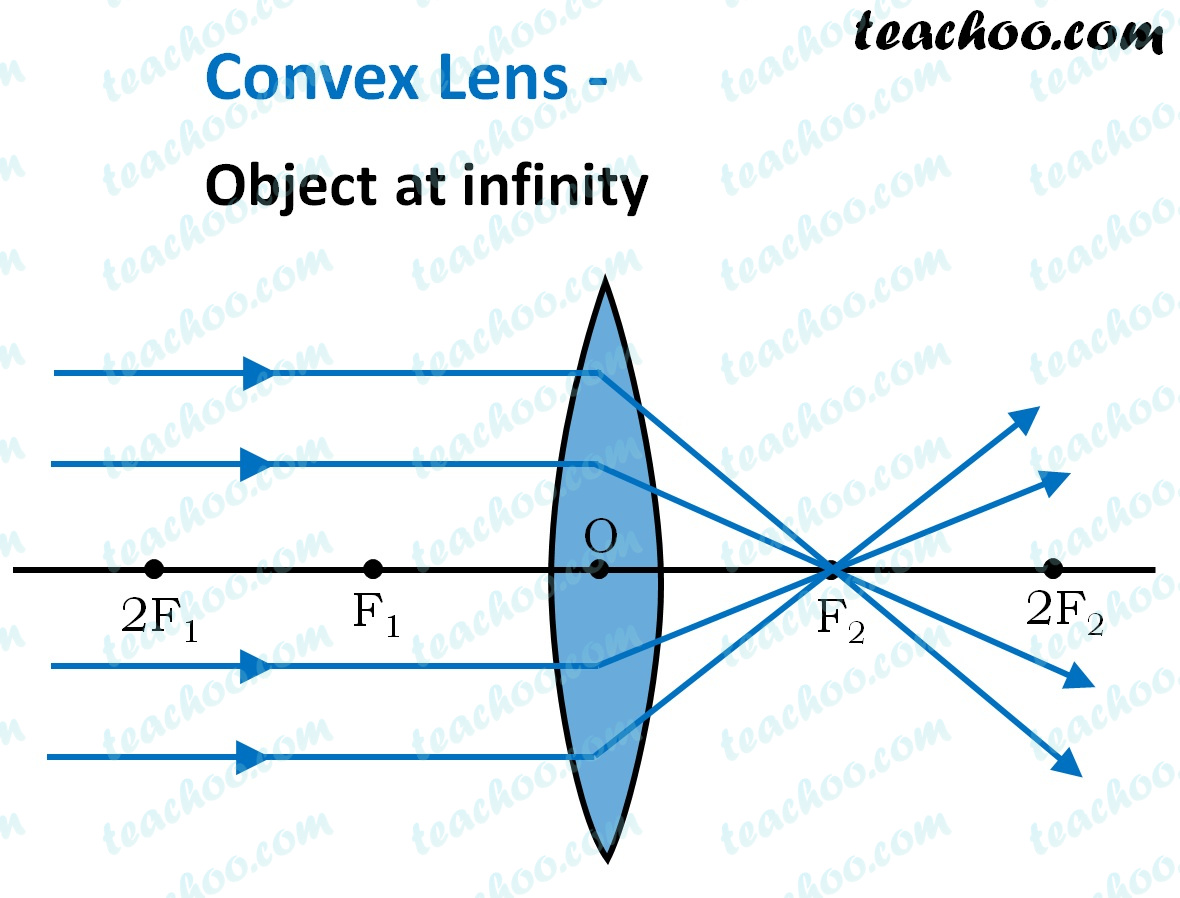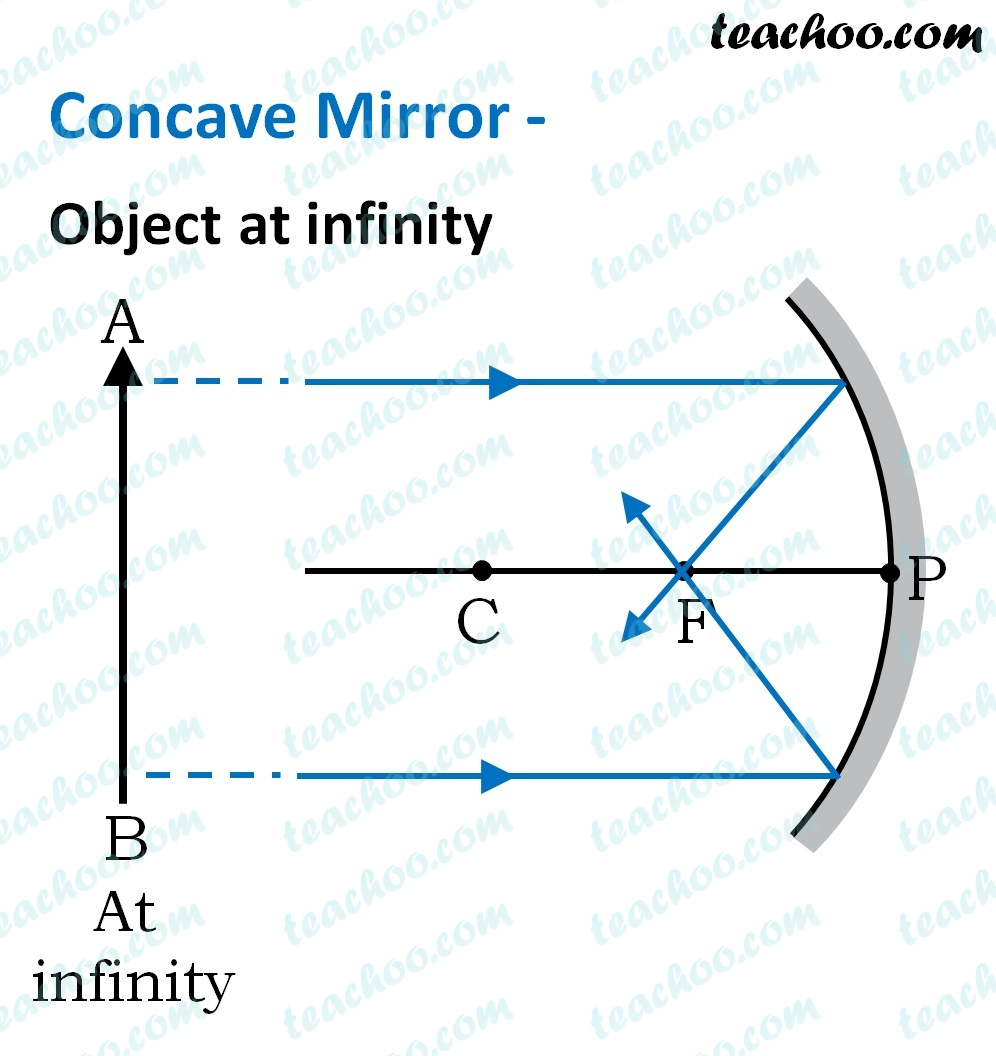MCQs from NCERT Exemplar

Class 10
Chapter 10 Class 10 - Light - Reflection and Refraction (Term 1)

## (d) Concave mirror as well as concave lens

Only converging lens and converging mirror can make a parallel beam of light incident on a point.

• Concave mirror is a converging mirror.
• Convex lenses converge to light rays, hence convex lenses are also called converging lenses .So, the correct answer is (a)